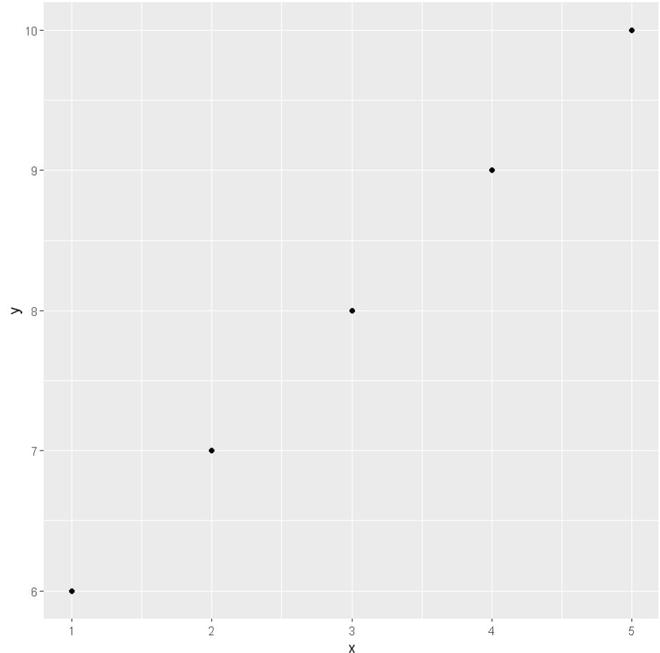# How to Fix: error: `mapping` must be created by `aes()` in R

• Last Updated : 20 Feb, 2022

In this article, we are going to see how to fix the error `mapping` must be created by `aes()` in R Programming Language.

Mapping must be created by aes() error occurs when we use aes() argument while creating a plot in ggplot2 with an incorrect place or without mapping syntax.

## Producing the Error

Here we will create dataframe with two variables and then try to plot with the incorrect place of aes().

## R

 `library``(ggplot2) ` ` `  `# Create example data ` `data <- ``data.frame``(x = 5:1,         ` `                   ``y = 10:6) ` `ggplot``() + ``geom_point``(data, ``aes``(x=x))`

Output:

`Error: `mapping` must be created by `aes()``

## Method 1: Solve using Mapping

We will deploy the mapping attributes in front of the aes() argument, this error occurs without using mapping syntax.

## R

 `library``(ggplot2) ` ` `  `# Create example data ` `data <- ``data.frame``(x = 5:1,         ` `                   ``y = 10:6) ` `ggplot``() + ``geom_point``(data, mapping=``aes``(x=x, y = y))`

Output:## Method 2: Solve using aes() method

We can also fix this error with using aes() in a right place, aes() argument within the ggplot() function.

## R

 `library``(ggplot2) ` ` `  `# Create example data ` `data <- ``data.frame``(x = 5:1,         ` `                   ``y = 10:6) ` `ggplot``(data, ``aes``(x=x, y)) + ``geom_point``()`

Output:My Personal Notes arrow_drop_up
Recommended Articles
Page :